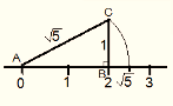Newbie

# Show how √5 can be represented on the number line. Q.3

• 0

Find the best solution of ncert class 9th chapter number systems how i solve the exercise 1.2 question number 3 find the easiest solution of the question also  find the best and simplest solution of this question.Show how √5 can be represented on the number line.

Share

1. Step 1: Let line AB be of 2 unit on a number line.

Step 2: At B, draw a perpendicular line BC of length 1 unit.

Step 3: Join CA

Step 4: Now, ABC is a right angled triangle. Applying Pythagoras theorem,

AB2+BC2 = CA2

22+12 = CA2 = 5

⇒ CA = √5 . Thus, CA is a line of length √5 unit.

Step 4: Taking CA as a radius and A as a center draw an arc touching

the number line. The point at which number line get intersected by

arc is at √5 distance from 0 because it is a radius of the circle

whose center was A.

Thus, √5 is represented on the number line as shown in the figure.• 0